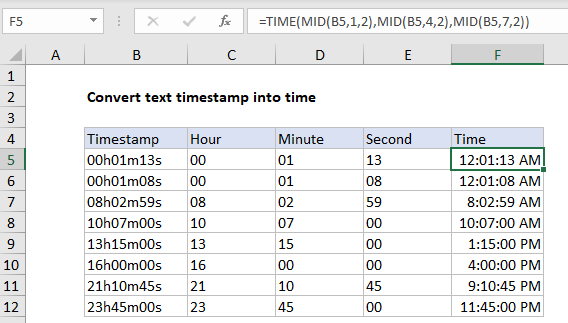## Excel Office

Excel How Tos, Tutorials, Tips & Tricks, Shortcuts

# Convert text timestamp into time in Excel

This tutorial show how to Convert text timestamp into time in Excel using the example below.

Top convert a timestamp entered as text into a proper Excel time, you can use the MID function to extract components and the TIME function to assemble the time.

## Formula

`=TIME(MID(A1,1,2),MID(A1,4,2),MID(A1,7,2))`## Explanation of how this formula works

In the example shown, the formula in F5 is:

`=TIME(MID(B5,1,2),MID(B5,4,2),MID(B5,7,2))`

This formula works for times entered in a particular format as shown below:

```00h01m13s
00h01m08s
08h02m59s```

Note the text string is always 9 characters long, and each component is 2 digits.

Worked Example:   How to extract text between parentheses in Excel

The core of this formula is the TIME function, which assembles a valid time using individual hour, minute, and second components. Since these values are all together in a single text string, the MID function is used to extract each component:

```MID(B5,1,2) // extract hour
MID(B5,4,2) // extract minute
MID(B5,7,2) // extract second```

The results are fed directly to the TIME function as arguments. The code below shows how the formula is solved in cell F5:

```=TIME(MID(B5,1,2),MID(B5,4,2),MID(B5,7,2))
=TIME("00","01","13")
=12:01:13```

Notice MID, as a text function, returns text instead of actual numbers. However, the TIME function still works properly, coercing the text values to numbers automatically.` `

Worked Example:   Extract word that begins with specific character in Excel